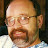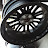Solve Equation Introduction Simplify Variable Expression 1 Tutor AlgebraSolve Equation Introduction Simplify Variable Expression 1 Tutor Algebra

This math video tutors and gives instruction about solving equations. The instruction is a brief and to the point teaching lesson on how to learn algebra and manipulate variables.
You can use properties of addition and multiplication to simplify expressions. Terms in an expression are like terms if they have identical variable parts. In a term that is the product of a number and a variable, the numerical part of the term is the coefficient of the variable. A term that is a number is called a constant term. The process of simplifying expressions with like terms is called combining like terms.

algebra tutors,algebra,college,combine,educational,equation,expression,for,ged,help,high,homework,how to,instruction,learn,lesson,like terms,linear,math,mathematics,online,practice,pre,property,school,simplify,solve,solving,teach,tutor,tutorial,tutoring,variable,video,with

algebratutors.org

2 Responses to “Solve Equation Introduction Simplify Variable Expression 1 Tutor Algebra”

1.Paul Bogdan says:

No, 6b + 2 is as simple as this gets (and is the answer). In order to get 8b we would have to have 6b + 2b (and we do not have this). You can only add 'like terms'. Like Terms are terms that are so similar that they can be added. In the original problem there were 3 terms and the b and the 5b were Like Terms because they are so similar that they can be added.

Robert: Please sit down one on one with your math teacher and get this cleared up right away. Ask me more questions when you get stuck.

2.Robert Legaspi says:

So would the answer be 8b?